# Learn The Science Behind The Time Value Of Money

By

on

I think that at some point in our lives we’ve heard or even said the clich saying ‘Time is Money’, without giving much thought to the real science behind the principle. Well in this post I would like to present some science that will give us all more depth on the concept, hopefully we can apply it appropriately.

Congratulations!!! You have won a cash prize! You have two payment options: A – Receive \$10,000 now OR B – Receive \$10,000 in three years. Which option would you choose?

What Is Time Value? If you’re like most people, you would choose to receive the \$10,000 now. After all, three years is a long time to wait. Why would any rational person defer payment into the future when he or she could have the same amount of money now? For most of us, taking the money in the present is just plain instinctive. So at the most basic level, the time value of money demonstrates that, all things being equal, it is better to have money now rather than later.

But why is this? A \$100 bill has the same value as a \$100 bill one year from now, doesn’t it? Actually, although the bill is the same, you can do much more with the money if you have it now because over time you can earn more interest on your money.

Back to our example: by receiving \$10,000 today, you are poised to increase the future value of your money by investing and gaining interest over a period of time. For Option B, you don’t have time on your side, and the payment received in three years would be your future value. To illustrate, we have provided a timeline: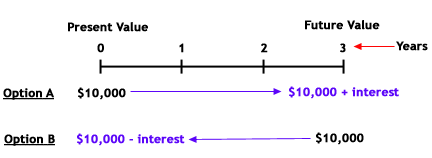If you are choosing Option A, your future value will be \$10,000 plus any interest acquired over the three years. The future value for Option B, on the other hand, would only be \$10,000. So how can you calculate exactly how much more Option A is worth, compared to Option B? Let’s take a look.

Future Value Basics: If you choose Option A and invest the total amount at a simple annual rate of 4.5%, the future value of your investment at the end of the first year is \$10,450, which of course is calculated by multiplying the principal amount of \$10,000 by the interest rate of 4.5% and then adding the interest gained to the principal amount:

 Future value of investment at end of first year: = (\$10,000 x 0.045) + \$10,000 = \$10,450

You can also calculate the total amount of a one-year investment with a simple manipulation of the above equation:

• Original equation: (\$10,000 x 0.045) + \$10,000 = \$10,450
• Manipulation: \$10,000 x [(1 x 0.045) + 1] = \$10,450
• Final equation: \$10,000 x (0.045 + 1) = \$10,450

The manipulated equation above is simply a removal of the like-variable \$10,000 (the principal amount) by dividing the entire original equation by \$10,000.

If the \$10,450 left in your investment account at the end of the first year is left untouched and you invested it at 4.5% for another year, how much would you have? To calculate this, you would take the \$10,450 and multiply it again by 1.045 (0.045 +1). At the end of two years, you would have \$10,920:

 Future value of investment at end of second year: = \$10,450 x (1+0.045) = \$10,920.25

The above calculation, then, is equivalent to the following equation:

 Future Value = \$10,000 x (1+0.045) x (1+0.045)

Think back to math class and the rule of exponents, which states that the multiplication of like terms is equivalent to adding their exponents. In the above equation, the two like terms are (1+0.045), and the exponent on each is equal to 1. Therefore, the equation can be represented as the following:We can see that the exponent is equal to the number of years for which the money is earning interest in an investment. So, the equation for calculating the three-year future value of the investment would look like this:This calculation shows us that we don’t need to calculate the future value after the first year, then the second year, then the third year, and so on. If you know how many years you would like to hold a present amount of money in an investment, the future value of that amount is calculated by the following equation: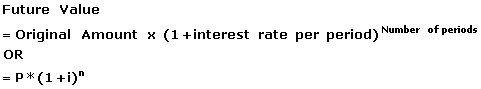Present Value Basics: If you received \$10,000 today, the present value would of course be \$10,000 because present value is what your investment gives you now if you were to spend it today. If \$10,000 were to be received in a year, the present value of the amount would not be \$10,000 because you do not have it in your hand now, in the present. To find the present value of the \$10,000 you will receive in the future, you need to pretend that the \$10,000 is the total future value of an amount that you invested today. In other words, to find the present value of the future \$10,000, we need to find out how much we would have to invest today in order to receive that \$10,000 in the future.

To calculate present value, or the amount that we would have to invest today, you must subtract the (hypothetical) accumulated interest from the \$10,000. To achieve this, we can discount the future payment amount (\$10,000) by the interest rate for the period. In essence, all you are doing is rearranging the future value equation above so that you may solve for P. The above future value equation can be rewritten by replacing the P variable with present value (PV) and manipulated as follows: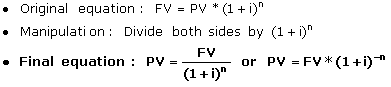Let’s walk backwards from the \$10,000 offered in Option B. Remember, the \$10,000 to be received in three years is really the same as the future value of an investment. If today we were at the two-year mark, we would discount the payment back one year. At the two-year mark, the present value of the \$10,000 to be received in one year is represented as the following:

 Present value of future payment of \$10,000 at end of year two:Note that if today we were at the one-year mark, the above \$9,569.38 would be considered the future value of our investment one year from now.

Continuing on, at the end of the first year we would be expecting to receive the payment of \$10,000 in two years. At an interest rate of 4.5%, the calculation for the present value of a \$10,000 payment expected in two years would be the following:

 Present value of \$10,000 in one year: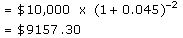Of course, because of the rule of exponents, we don’t have to calculate the future value of the investment every year counting back from the \$10,000 investment at the third year. We could put the equation more concisely and use the \$10,000 as FV. So, here is how you can calculate today’s present value of the \$10,000 expected from a three-year investment earning 4.5%: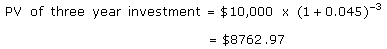So the present value of a future payment of \$10,000 is worth \$8,762.97 today if interest rates are 4.5% per year. In other words, choosing Option B is like taking \$8,762.97 now and then investing it for three years. The equations above illustrate that Option A is better not only because it offers you money right now but because it offers you \$1,237.03 (\$10,000 – \$8,762.97) more in cash! Furthermore, if you invest the \$10,000 that you receive from Option A, your choice gives you a future value that is \$1,411.66 (\$11,411.66 – \$10,000) greater than the future value of Option B.

Present Value of a Future Payment: Let’s add a little spice to our investment knowledge. What if the payment in three years is more than the amount you’d receive today? Say you could receive either \$15,000 today or \$18,000 in four years. Which would you choose? The decision is now more difficult. If you choose to receive \$15,000 today and invest the entire amount, you may actually end up with an amount of cash in four years that is less than \$18,000. You could find the future value of \$15,000, but since we are always living in the present, let’s find the present value of \$18,000 if interest rates are currently 4%. Remember that the equation for present value is the following: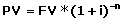In the equation above, all we are doing is discounting the future value of an investment. Using the numbers above, the present value of an \$18,000 payment in four years would be calculated as the following:

 Present ValueFrom the above calculation we now know our choice is between receiving \$15,000 or \$15,386.48 today. Of course we should choose to postpone payment for four years!

The Bottom Line
These calculations demonstrate that time literally is money – the value of the money you have now is not the same as it will be in the future and vice versa. So, it is important to know how to calculate the time value of money so that you can distinguish between the worth of investments that offer you returns at different times.

Investopedia: Shauna Carther